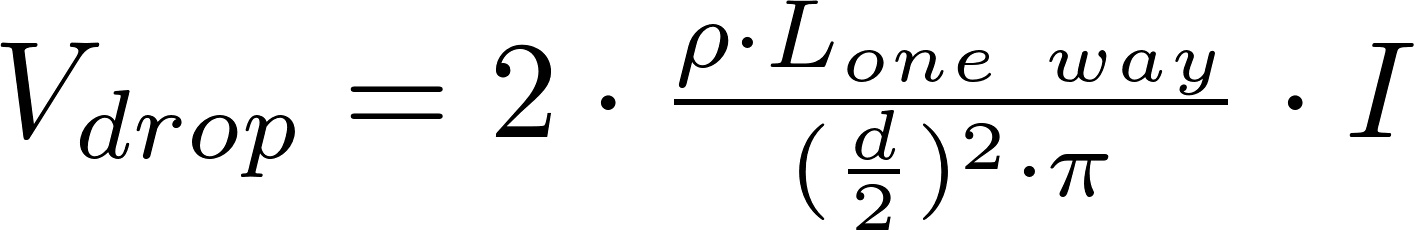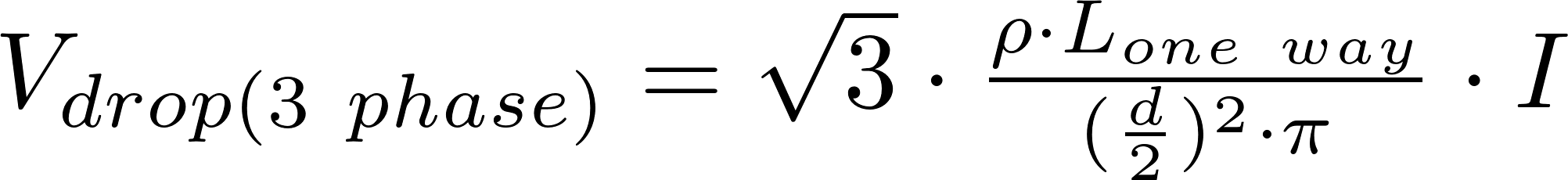# Voltage drop

The voltage drop is the voltage that is lost in a wire due to its resistance. The voltage drop can be decreased by using shorter or thicker wire, lowering the current or using a metal with a lower resistivity.

### FormulasV is the symbol for voltage and is measured in volt (V).
ρ is the symbol for resistivity and is measured in ohm-meter (Ω⋅m).
l is the symbol for length and is measured in meter (m).
I is the symbol for current and is measured in ampere (A).

### Resistivity

Resistivity is the property of a metal to resist the flow of current. The resistivity is different for each material and it is measured in ohm-meter (Ω⋅m).

material resistivity ρ 10⁻⁹Ω⋅m
silver 15.9
copper 16.8
aluminium 26.5
tungsten 56
iron 97.1
platinum 106
manganin 482